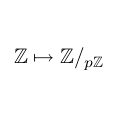CGAL 5.1.3 - Modular Arithmetic
Modular Arithmetic ReferenceMichael Hemmer and Sylvain Pion
This package provides arithmetic over finite fields. The provided tools are in particular useful for filters based on modular arithmetic and algorithms based on Chinese remainder.
Introduced in: CGAL 3.4
BibTeX: cgal:h-ma-21a

## Concepts

• Modularizable
• ModularTraits
• ModularTraits::ModularImage
• ModularTraits::ModularImageRepresentative

## Types

• CGAL::Residue
• CGAL::Modular_traits<T>

Concepts

## Classes

class  CGAL::Modular_traits< T >
An instance of Modular_traits is a model of ModularTraits, where T is the associated type. More...

class  CGAL::Residue
The class Residue represents a finite field $$\mathbb{Z}{/p\mathbb{Z}}$$, for some prime number $$p$$. More...